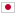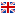Contest Duration: - (local time) (300 minutes) Back to Home
C - Ninja Map/Time Limit: 2 sec / Memory Limit: 512 MB

### Problem Statement

Intersections of Crossing Path City are aligned to a grid. There are $N$ east-west streets which are numbered from 1 to $N$, from north to south. There are also $N$ north-south streets which are numbered from 1 to $N$, from west to east. Every pair of east-west and north-south streets has an intersection; therefore there are $N^2$ intersections which are numbered from 1 to $N^2$.

Surprisingly, all of the residents in the city are Ninja. To prevent outsiders from knowing their locations, the numbering of intersections is shuffled.

You know the connections between the intersections and try to deduce their positions from the information. If there are more than one possible set of positions, you can output any of them.

### Input

The input consists of a single test case formatted as follows.

$N$ $a_1 \ b_1$ $\cdots$ $a_{2N^2-2N} \ b_{2N^2-2N}$

The first line consists of an integer $N \ (2 \le N \le 100)$. The following $2N^2-2N$ lines represent connections between intersections. The $(i+1)$-th line consists of two integers $a_i$ and $b_i$ ($1 \le a_i, b_i \le N^2$, $a_i \ne b_i$), which represent that the $a_i$-th and $b_i$-th intersections are adjacent. More precisely, let's denote by $(r, c)$ the intersection of the $r$-th east-west street and the $c$-th north-south street. If the intersection number of $(r, c)$ is $a_i$ for some $r$ and $c$, then the intersection number of either $(r - 1, c)$, $(r + 1, c)$, $(r, c - 1)$ or $(r, c + 1)$ must be $b_i$. All inputs of adjacencies are different, i.e., $(a_i,b_i)\ne(a_j,b_j)$ and $(a_i,b_i)\ne(b_j,a_j)$ for all $1 \le i < j \le 2N^2-2N$. This means that you are given information of all adjacencies on the grid.

The input is guaranteed to describe a valid map.

### Output

Print a possible set of positions of the intersections. More precisely, the output consists of $N$ lines each of which has space-separated $N$ integers. The $c$-th integer of the $r$-th line should be the intersection number of $(r, c)$.

If there are more than one possible set of positions, you can output any of them.

### Sample Input 1

3
1 2
4 7
8 6
2 3
8 9
5 3
4 6
5 6
7 8
1 4
2 6
5 9


### Output for Sample Input 1

7 4 1
8 6 2
9 5 3


The following output will also be accepted.

1 2 3
4 6 5
7 8 9


### Sample Input 2

4
12 1
3 8
10 7
13 14
8 2
9 12
6 14
11 3
3 13
1 10
11 15
4 15
4 9
14 10
5 7
2 5
6 1
14 5
16 11
15 6
15 13
9 6
16 4
13 2


### Output for Sample Input 2

8 2 5 7
3 13 14 10
11 15 6 1
16 4 9 12